# NCERT Solutions class 12 Chemistry Chemical kinetics part 2## myCBSEguide App

CBSE New Sample Papers 2021

CBSE Board Exam Papers, Question Bank, NCERT Solutions, NCERT Exemplars, Revison Notes, Free Videos, CBSE Papers, MCQ Tests & more.

NCERT Solutions class 12 Chemistry Chemical kinetics part 2 Class 12 Chemistry book solutions are available in PDF format for free download. These ncert book chapter wise questions and answers are very helpful for CBSE board exam. CBSE recommends NCERT books and most of the questions in CBSE exam are asked from NCERT text books. Class 12 Chemistry chapter wise NCERT solution for Chemistry part 1 and Chemistry part 2 for all the chapters can be downloaded from our website and myCBSEguide mobile app for free.

Download   NCERT solutions for Chemical kinetics part 2 as PDF.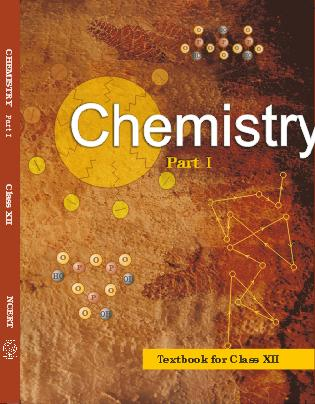## NCERT Class 12 Chemistry Chapter-wise Solutions

•  1 – The Solid State
• 2 – Solutions
• 3 – Electrochemistry
• 4 – Chemical Kinetics
• 5 – Surface Chemistry
• 6 – General Principles and Processes of Isolation of Elements
• 7 – The p-Block Elements
• 8 – The d-and f-Blocks Elements
• 9 – Coordination Compounds
• 10 – Haloalkanes and Haloarenes
• 11 – Alcohols, Phenols and Ethers
• 12 – Aldehydes, Ketones and Carboxylic Acids
• 13 – Amines
• 14 – Biomolecules
• 15 – Polymers
• 16 – Chemistry in Everyday Life

## CHAPTER FOUR CHEMICAL KINETICS

• 4.1 Rate of a Chemical Reaction
• 4.2 Factors Influencing Rate of a Reaction
• 4.3 Integrated Rate Equations
• 4.4 Pseudo First Order Reaction
• 4.5 Temperature Dependence of the rate of a Reaction
• 4.6 Collision Theory of Chemical Reactions

## NCERT Solutions class 12 Chemistry Chemical kinetics part 2

16. The rate constant for a first order reaction is 60 s-1. How much time will it take to reduce the initial concentration of the reactant to its 1/16th value?

17. During nuclear explosion, one of the products is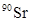with half-life of 28.1 years. If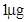ofwas absorbed in the bones of a newly born baby instead of calcium, how much of it will remain after 10 years and 60 years if it is not lost metabolically.

18. For a first order reaction, show that time required for 99% completion is twice the time required for the completion of 90% of reaction.

19. A first order reaction takes 40 min for 30% decomposition. Calculate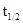.

20. For the decomposition of azoisopropane to hexane and nitrogen at 543 K, the following data are obtained.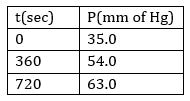Calculate the rate constant.

21. The following data were obtained during the first order thermal decomposition of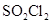at a constant volume.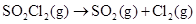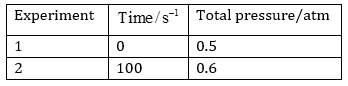Calculate the rate of the reaction when total pressure is 0.65 atm.

22. The rate constant for the decomposition of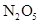at various temperatures is given below: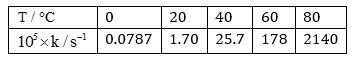Draw a graph between ln k and 1/T and calculate the values of A and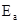. Predict the rate constant at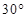and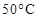.

23. The rate constant for the decomposition of hydrocarbons is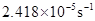at 546 K. If the energy of activation is 179.9 kJ/mol, what will be the value of pre-exponential factor.

24. Consider a certain reaction A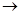Products with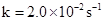. Calculate the concentration of A remaining after 100 s if the initial concentration of A is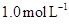.

25. Sucrose decomposes in acid solution into glucose and fructose according to the first order rate law, with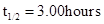. What fraction of sample of sucrose remains after 8 hours?

26. The decomposition of hydrocarbon follows the equation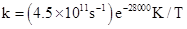.

Calculate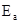.

27. The rate constant for the first order decomposition of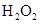is given by the following equation: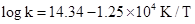Calculatefor this reaction and at what temperature will its half-period be 256 minutes?

28. The decomposition of A into product has value of k as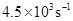at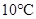and energy of activation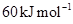. At what temperature would k be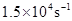?

29. The time required for 10% completion of a first order reaction at 298 K is equal to that required for its 25% completion at 308 K. If the value of A is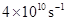. Calculate k at 318 K and.

30. The rate of a reaction quadruples when the temperature changes from 293 K to 313 K. Calculate the energy of activation of the reaction assuming that it does not change with temperature.

## NCERT Solutions class 12 Chemistry

NCERT Solutions Class 12 Chemistry PDF (Download) Free from myCBSEguide app and myCBSEguide website. Ncert solution class 12 Chemistry includes text book solutions from both part 1 and part 2. NCERT Solutions for CBSE Class 12 Chemistry have total 16 chapters. 12 Chemistry NCERT Solutions in PDF for free Download on our website. Ncert Chemistry class 12 solutions PDF and Chemistry ncert class 12 PDF solutions with latest modifications and as per the latest CBSE syllabus are only available in myCBSEguide NCERT Solutions class 12 Chemistry Amines part 1

## CBSE app for Class 12

To download NCERT Solutions for class 12 Chemistry, Physics, Biology, History, Political Science, Economics, Geography, Computer Science, Home Science, Accountancy, Business Studies and Home Science; do check myCBSEguide app or website. myCBSEguide provides sample papers with solution, test papers for chapter-wise practice, NCERT solutions, NCERT Exemplar solutions, quick revision notes for ready reference, CBSE guess papers and CBSE important question papers. Sample Paper all are made available through the best app for CBSE students and myCBSEguide website.